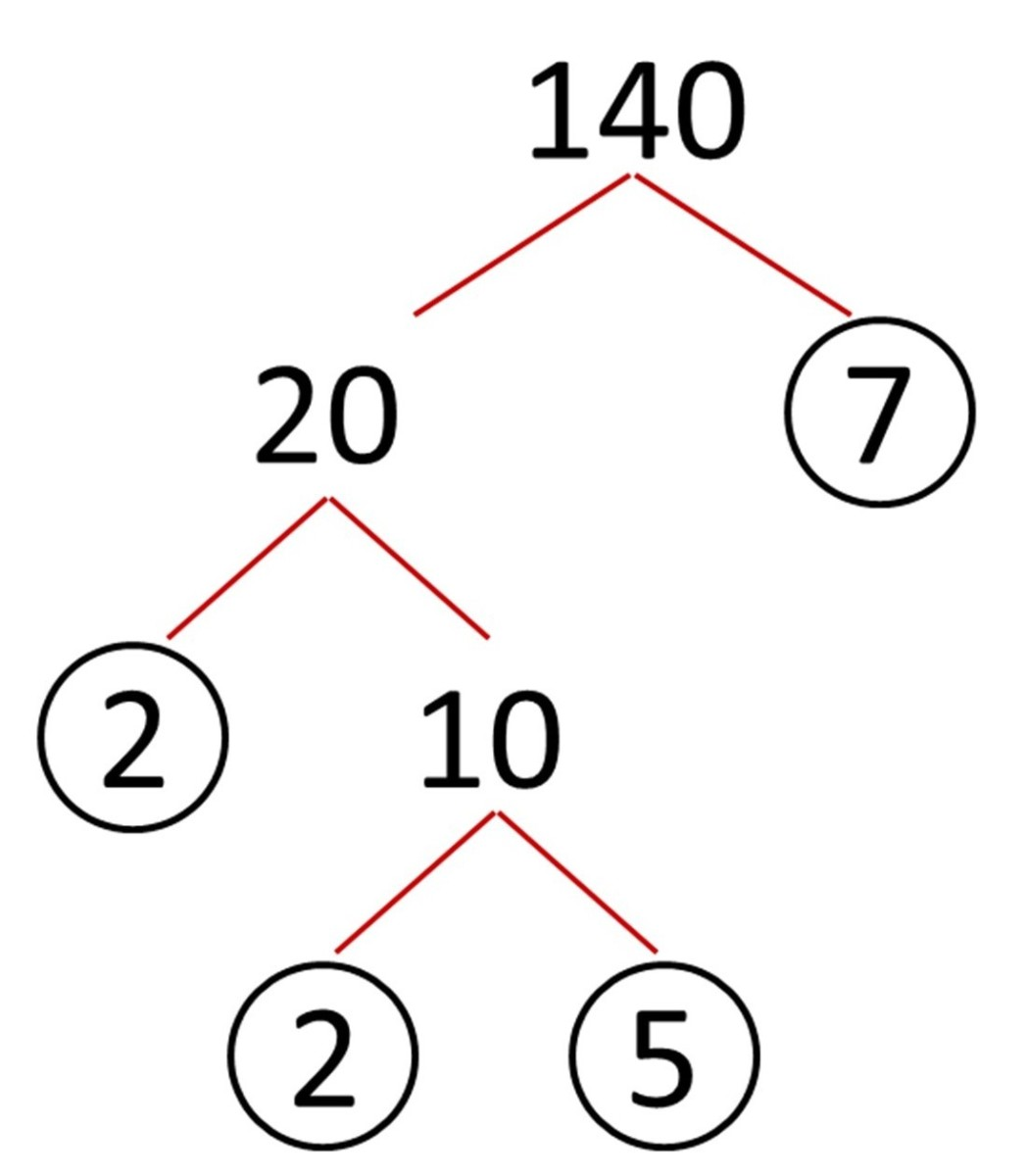# How to Write a Number as a Product of Its Prime Factors

• Author:
• Updated date:

I am a former maths teacher and owner of DoingMaths. I love writing about maths, its applications and fun mathematical facts.

## What Are Prime Numbers?

Before we can look at prime factors, we must first look at what a prime number is.

The prime numbers are defined as any number with exactly two factors (numbers which will divide into it perfectly with no remainder).

The number 2 is a prime number as it has only two factors, 1 and 2. 5 is also a prime number as it has exactly two factors, 1 and 5. In fact, for any prime number, its two factors must be 1 and itself.

On the other hand, the number 6 is not a prime number as it can be divided by 1, 2, 3 and 6 for a total of four factors. 1 is also not prime as it has only one factor; itself.

## What Are Prime Factors?

As the name suggests, the prime factors of a number are the factors of a number which are also prime. For example, the number 20 has six factors; 1, 2, 4, 5, 10 and 20. Of these factors, 2 and 5 are the only prime numbers, hence we call these the prime factors of 20.

When writing a number as a product of its prime factors, we want to rewrite the number as the multiplication of prime factors which will get us back to this number. For example 20 = 2 × 2 × 5. We have three numbers, all of which are prime, which multiply together to make 20.

## Using a Factor Tree for Prime Factorisation

When numbers have multiple factors, it can be tricky to find all of the prime factors. Furthermore, many numbers have repeated prime factors, such as in the example above where we saw that we used 2 twice to get 20. These can be especially difficult to spot.

One way to make sure that we find every prime factor needed is to use a prime factor tree. This works by taking our original number and breaking it down into a pair of factors that multiply together to give the original number. If either of these is prime, we leave it there. If either number can be broken down again, we do so. We keep repeating this until we can go no further, at which point we will have every prime factor.

## Example - Write 140 as the Product of Its Prime Factors

The first step is to find two numbers that multiply together to make 140. There are multiple pairs that will do this e.g. 2 × 70, 4 × 35, 5 × 28 etc, but it doesn't matter which one we choose. We will find out more about the reason why later on.

In this example, let's start with 10 × 14. To write this we start with 140 at the top and have two branches coming down to our two factors as in the picture below.

Scroll to Continue

## Continuing the Prime Factorisation of 140

Now that we have our first pair of factors, we look at each factor in turn and split them up further if we can.

On the left we have 10. This is equal to 2 × 5, so we split this along two branches to the 2 and 5. On the right we have 14 which is equal to 2 × 7 and so we split this accordingly. The four factors which we have now are all prime, so we circle them to denote this and cannot split any further.

## Writing the Prime Factors as a Product

Our prime factor tree for 140 has four branch ends where we have a prime number and hence cannot split the factors any further. These are 2, 5, 2 and 7. Remembering that each pairing multiplies to make the number above, we can see that 140 must equal the product of these four prime numbers.

So we have 140 = 2 × 2 × 5 × 7 = 22 × 5 × 7

## Can We Get Different Prime Factors?

Thinking back to our original split of the number 140, we had several options for pairs of factors. It might be tempting to think that if we had chosen a different starting pair, we would then end up with a different answer, but this isn't the case.

Look at the image below. In this case we start with 20 × 7. We circle 7 as this is prime, but continue to split 20. When we complete the tree we end up with the same four prime numbers as before; 2, 2, 5 and 7.Prime factorisation tree for 140 - different starting pair.My own image

## Unique Factorisation - The Fundamental Theorem of Arithmetic

In fact, it doesn't matter which order we choose to split up 140 or any other number into pairs of factors; we will always end up with the same prime factors at the end.

This is formally known as the Fundamental Theorem of Arithmetic which states that every integer larger than 1 can be written as a unique product of prime factors.

For example, 140 = 22 × 5 × 7 as we have already seen and cannot be written as a combination of any other primes.

1100 = 22 × 52 × 11 and again cannot be written as a product of any different primes or using different powers.